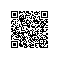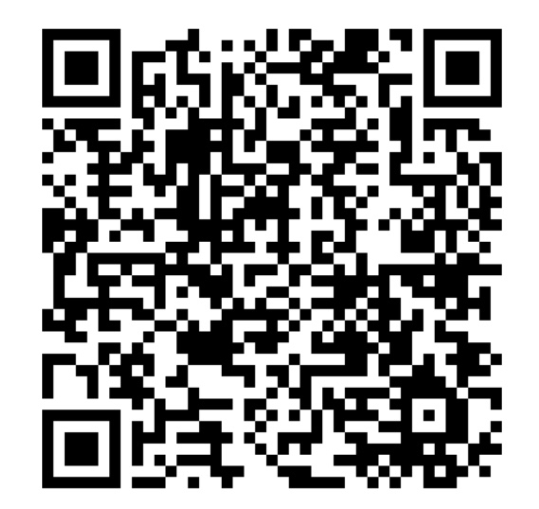# 杨老师课堂_VBA学习教程之一键合并所有文件

要求：在C盘中有一个文件夹，文件夹中有文件存在

Sub wjhb()
Dim str As String
Dim wb As Workbook

str = Dir("c:\data\*.*")

For i = 1 To 20
' 1 打开文件
Set wb = Workbooks.Open("c:\data\" & str & "")
' 2 从遍历中的某一个工作簿中的第一张表执行拷贝 到这个工作簿中的最后一张表中去
wb.Sheets(1).Copy after:=ThisWorkbook.Sheets(ThisWorkbook.Sheets.Count)
' 3 在这个工作簿中的最后一张表的名字是【已经完成拷贝操作】  = 文件名称
ThisWorkbook.Sheets(ThisWorkbook.Sheets.Count).Name = Split(str, ".")(0)
'4 遍历中的工作簿执行关闭
wb.Close
'下一次遍历
str = Dir

If str = "" Then
Exit For
End If

Next

End Sub

End Sub
Sub wjhb2()
Dim str As String
Dim wb As Workbook

str = Dir("c:\data\*.xlsx")

For i = 1 To 20

Set wb = Workbooks.Open("c:\data\" & str & "")

wb.Sheets(1).Copy after:=ThisWorkbook.Sheets(ThisWorkbook.Sheets.Count)
ThisWorkbook.Sheets(ThisWorkbook.Sheets.Count).Name = str
wb.Close
str = Dir

If str = "" Then
Exit For
End If

Next
End Sub使用钉钉扫一扫加入圈子
+ 订阅